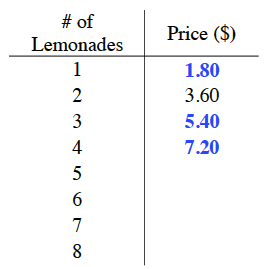Home > CC2 > Chapter 4 > Lesson 4.2.1 > Problem4-29

4-29.

The lemonade stand at the county fair sells the lemonade at a price of two cups for $\3.60$.  Complete the table at right to find what Paula’s family will pay to buy lemonade for all eight members of the family.  Homework Help ✎

 # of Lemonades Price (\$) $1$ $2$ $3.60$ $3$ $4$ $5$ $6$ $7$ $8$

Since $2$ cups of lemonade is twice as much as $1$ cup of lemonade, to find the price of $1$ cup of lemonade, you must find half of the price of $2$ cups of lemonade.

You can also solve the problem by setting up a proportion like the one below, then solve for $x$.

$\large\frac{\text{2 cups of lemonade}}{\text{3.60 dollars}} \left( \frac{?}{?} \right) = \frac{\text{1 cup of lemonade}}{x\text{ dollars}}$

To find the price of any amount of lemonade, in the equation above substitute ''$1$ cup of lemonade'' with the number of lemonades you are looking for.